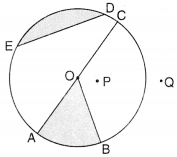NCERT Solutions for Class 6 Maths Chapter 4 Basic Geometrical Ideas Ex 4.6 are part of NCERT Solutions for Class 6 Maths. Here we have given NCERT Solutions for Class 6 Maths Chapter 4 Basic Geometrical Ideas Ex 4.6.

 Board CBSE Textbook NCERT Class Class 6 Subject Maths Chapter Chapter 4 Chapter Name Basic Geometrical Ideas Exercise Ex 4.6 Number of Questions Solved 4 Category NCERT Solutions

## NCERT Solutions for Class 6 Maths Chapter 4 Basic Geometrical Ideas Ex 4.6

Question 1.
From the figure identify
(a) the center of the circle
(c) a diameter
(d) a chord(e) two points in the interior
(f) a point in the exterior
(g) a sector
(h) a segment.
Solution :
(a) O is the centre of the circle.
(b) $$\bar { OA }$$, $$\bar { OB }$$, $$\bar { OC }$$ are three radii of the circle.
(c) $$\bar { AC }$$ is a diameter of the circle.
(d) $$\bar { ED }$$ is a chord of the circle,
(e) O and P are two points in the interior.
(f) Q is a point in the exterior.
(e) OAB (shaped portion) is a sector of the circle.
(f) Shaded portion of the circular region enclosed by line segment ED and the corresponding arc.

Question 2.
(a) Is every diameter of a circle also a chord?
(b) Is every chord of a circle also a diameter?
Solution :
(a) Yes! every diameter of a circle is also a chord.
(b) No ! every chord of a circle is not also a

Question 3.
Draw any circle and mark:
(a) it’s center
(c) a diameter
(d) a sector
(e) a segment
(f) a point in its interior
(g) a point in its exterior
(h) an arc.
Solution :
(a) O is the centre.
(b) $$\bar { OA }$$ is a radius.
(c) $$\bar { AB }$$ is a diameter.
(d) OBC is a sector.
(e) AGD is a segment.
(f) P is a point in its interior.
(g) Q is a point in its exterior.(h) $$\hat { EF }$$  is an arc.

Question 4.
Say true or false:
(a) Two diameters of a circle will necessarily intersect.
(b) The center of a circle is always in its interior.
Solution :
(a) True
(b) True.

We hope the NCERT Solutions for Class 6 Maths Chapter 4 Basic Geometrical Ideas Ex 4.6 help you. If you have any query regarding NCERT Solutions for Class 6 Maths Chapter 4 Basic Geometrical Ideas Ex 4.6, drop a comment below and we will get back to you at the earliest.